# Nonagon

A nonagon is a polygon that has nine sides. In the figure below are several types of nonagons.

## Nonagon classifications

Like other polygons, a nonagon can be classified as regular or irregular. If all the sides and interior angles of a nonagon are equal, it is a regular nonagon. Otherwise it is an irregular nonagon.

Regular nonagonIrregular nonagon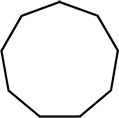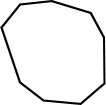All sides and interior angles are equal Not all sides and angles are equal

Nonagons and other polygons can also be classified as either convex or concave. If all interior angles of a nonagon are less than 180°, it is convex. If one or more interior angles is larger than 180°, it is concave. A regular nonagon is a convex nonagon.

Convex nonagonConcave nonagon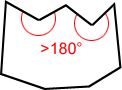All interior angles < 180° One or more interior angles > 180°

## Diagonals of nonagon

A diagonal is a line segment joining two non-consecutive vertices. A total of twenty-seven distinct diagonals can be drawn for a nonagon. The following figure is an example.

There are 6 diagonals extending from each of the 9 vertices of the nonagon above creating a total of 27 diagonals.

## Internal angles of a nonagon

The sum of the interior angles of a nonagon equals 1260°.

As shown in the figure above, six diagonals can be drawn to divide the nonagon into seven triangles. The blue lines above show just one way to divide the nonagon into triangles; there are others. The sum of interior angles of the seven triangles equals the sum of interior angles of the nonagon. Since the sum of the interior angles of a triangle is 180°, the sum of the interior angles of the nonagon is 9 × 180° = 1260°.

## Regular nonagon

A regular nonagon is a nonagon in which all sides have equal length and all interior angles have equal measure.

### Angles of a regular nonagon

Since each of the nine interior angles in a regular nonagon are equal in measure, each interior angle measures 1260° ÷ 9 = 140°, as shown below.

Each exterior angle of a regular nonagon has an equal measure of 40°.

### Symmetry in a regular nonagon

A regular nonagon has 9 lines of symmetry and a rotational symmetry of order 9, meaning that it can be rotated in such a way that it will look the same as the original shape 9 times in 360°.

Lines of symmetryRotational symmetry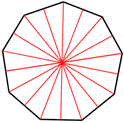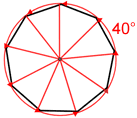9 lines of symmetry Nine 40° of rotation about the center.